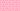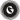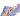PricingResources

# Standard Deviation in FinanceWritten by

This statistical term might sound complicated, but standard deviation is frequently used by investors to determine how risky a stock or fund is. In a nutshell, it shows you how much a fund’s returns vary from the average. Learn more about working out standard deviation in finance with our guide below.

## What is standard deviation?

To understand how this concept is applied in the world of finance, it first helps to answer the question of ‘what is standard deviation’, statistically speaking. In statistics, imagine that you’re looking at a series or numbers or dataset. Its standard deviation is a measure of how high or low the values go in relation to the set’s average or mean.

## How standard deviation works in finance

Standard deviation is used to measure overall market volatility, showing how widely a fund’s prices swing from the average. It can also be applied to individual stock performance. If a set of returns is all over the map, this means that the investment in question is riskier, because its returns can’t be easily predicted.

You can calculate standard deviation by measuring each data point’s deviation in relation to the mean.

• High deviation: the data points are spread out far apart from the mean, indicating high volatility.

• Low deviation: the data points stick closely to the set’s mean, indicating low volatility.

The more widespread the data points are, the higher its standard deviation – and greater the investment risk. Here’s what all this means when applied to typical market fluctuations:

• As prices become increasingly volatile, standard deviation rises. When prices calm down, standard deviation is lower.

• Market tops with increased volatility over a short-term period indicates that traders are feeling nervous.

• Market tops with decreasing volatility over a long-term period indicates a bull market.

• Market bottoms that come with decreased volatility over a long-term period indicate that traders are disinterested.

• Market bottoms that grow increasingly volatile over a short-term period indicate that traders are panic selling.

As you can see, looking at standard deviation is useful for investors as a gauge of trader sentiment, and can be used as one predictor of where the market’s headed.

## Standard deviation formula

Working out standard deviation is easy using a standard deviation formula like the following:

There are plenty of online calculators that will apply the formula for you. Step by step, you can also calculate the standard deviation as follows:

1. Calculate mean value by adding together all data points in the set and dividing them by the total number of data points.

2. Calculate the variance for each data point by subtracting the mean value from the data point value.

3. Square each resulting variance and add the points together. Divide this from the number of data points minus one.

4. Take the square root of the variance to find standard deviation.

## Standard deviation example and limitations

Imagine that you’re looking at the performance of a mutual fund that gained 1.5% every single month over the past 12 months. It would have a standard variation of zero for this time period, because there is no variance from the mean.

As another standard deviation example, if this same fund lost 1.5% each month over the same period, it would also have an SV of zero. Although it predictably lost money, it didn’t vary from the mean.

On the other hand, imagine a similar mutual fund that gained 10% one month and 25% the next, before losing 6% in the third month, and so on. Its standard deviation would be much higher to reflect this volatility.

It’s important to remember that standard deviation only shows you how volatile an investment is; it can’t make any value judgments. A fund that loses money at a steady rate will have a low standard deviation, but that doesn’t make it a good investment. This measure will always be impacted by outlying, extreme values, but not all uncertainty is risk. Above-average returns will also show up as high standard deviation, so be sure you’re looking at the big picture.

#### We can help

GoCardless helps you automate payment collection, cutting down on the amount of admin your team needs to deal with when chasing invoices. Find out how GoCardless can help you with ad hoc payments or recurring payments.Contact sales

Sales

Support